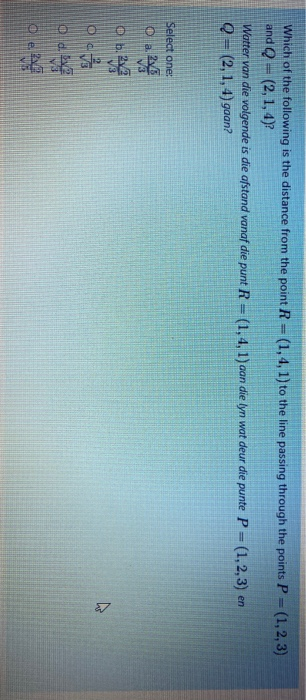# Which of the following is the distance from the point R = (1,4, 1) to the line passing through the points P = (1,2,3) and Q = (2, 1, 4)? | %3D Watter van die volgende is die afstand vanaf die punt R = (1,4, 1) aan die lyn wat deur die punte P = (1,2,3) en %3D Q= (2, 1, 4) gaan? Select one: O a 2 Ob. 2 O d. e.

Questionhelp_outlineImage TranscriptioncloseWhich of the following is the distance from the point R = (1,4, 1) to the line passing through the points P = (1,2,3) and Q = (2, 1, 4)? | %3D Watter van die volgende is die afstand vanaf die punt R = (1,4, 1) aan die lyn wat deur die punte P = (1,2,3) en %3D Q= (2, 1, 4) gaan? Select one: O a 2 Ob. 2 O d. e. fullscreen

### Want to see this answer and more?

Experts are waiting 24/7 to provide step-by-step solutions in as fast as 30 minutes!*

*Response times may vary by subject and question complexity. Median response time is 34 minutes for paid subscribers and may be longer for promotional offers.
Tagged in
Math